# Four integers

Fnd four consecutive integers so that the product of the first two is 70 times smaller than the product of the next two.

a =  16
b =  17
c =  18
d =  19

### Step-by-step explanation:

$b=a+1=16+1=17$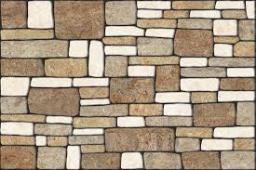Did you find an error or inaccuracy? Feel free to write us. Thank you!Tips to related online calculators
Looking for help with calculating roots of a quadratic equation?
Do you have a linear equation or system of equations and looking for its solution? Or do you have a quadratic equation?

## Related math problems and questions:

• Diofant 2Is equation 70x +52y = 34 solvable on the set of integers Z?
• Three numbersFind three numbers so that the second number is 4 times greater than the first and the third is lower by 5 than the second number. Their sum is 67.
• The sum 8The sum of two numbers is 21. If three times the smaller numbers is two less than twice the larger number, find the two numbers.
• Digit sumThe digit sum of the two-digit number is nine. When we turn figures and multiply by the original two-digit number, we get the number 2430. What is the original two-digit number?
• Two unknown numbersOne number is 2 less than a second number. Twice the second number is 14 less than 5 times the first. Find the two numbers.
• Two numbersOne number is by 79 larger than the other. If we divide the larger number by the smaller one, we get the ratio 5 and the remainder 11. Determine both numbers.
• Three ints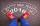The sum of three consecutive integers is 2016. What numbers are they?
• Find twoFind two consecutive natural numbers whose product is 1 larger than their sum. Searched numbers expressed by a fraction whose numerator is the difference between these numbers and the denominator is their sum.
• n-gon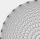Gabo draws n-gon, which angles are consecutive members of an arithmetic sequence. The smallest angle is 70° biggest 170°. How many sides have Gabo's n-gon?
• Two-digit numberIn a two-digit number, the number of tens is three greater than the number of units. If we multiply the original number by a number written in the same digits but in reverse order, we get product 3 478. Find the original number.
• In the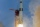In the arithmetic sequence a1 = 4.8, d = 0.4. How many consecutive members, starting with the first, need to be added so that the sum is greater than 170?
• FlowerbedWe enlarge the circular flower bed, so its radius increased by 3 m. The substrate consumption per enlarged flower bed was (at the same layer height as before magnification) nine times greater than before. Determine the original flowerbed radius.
• The largerThe larger of two numbers is nine more than four times the smaller number. The sum of the two numbers is fifty-nine. Find the two numbers.
• Sales of productsFor 80 pieces of two quality products a total sales is 175 Eur. If the first quality product was sold for n EUR per piece (n natural number) and the second quality product after 2 EUR per piece. How many pieces of the first quality were sold?
• Three friends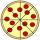Divide 570 euros to three friends so that first will get 50 euros less than the second and third twice more than the first. How many euros will get everyone?
• The sumThe sum of the squares of two immediately following natural numbers is 1201. Find these numbers.
• Odd numbersThe sum of four consecutive odd numbers is 1048. Find those numbers ...